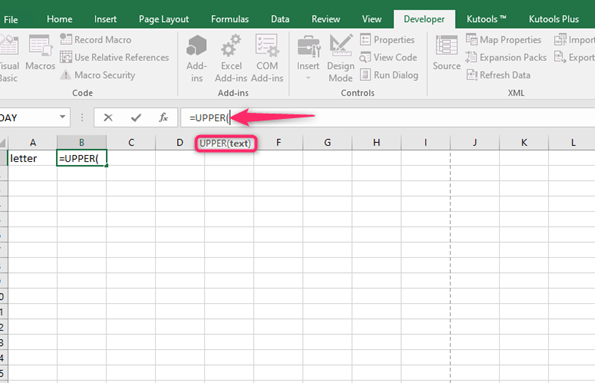# How to Capitalize the First Letter of a Text String in Excel

Excel can be used with text data apart from numerical data. You could use it to record a business's names, goods, or services. The test data should be made by capitalizing the first letters of all the words in the cells. You may want to capitalize the first letter of each word or only the first letter of the first word. We shall cover some of the methods used to capitalize the first letters of words in Excel cells.

## Capitalizing the first letter using formulas

How you can "capitalize the first letter" of each word

The formula to use is =PROPER(A2)Using this formula will make the first letter of each word capital

### How to capitalize only the first letter of the first word

Since there is no straightforward formula to capitalize only the first letter of the first word, there are two methods to do this.

How you can "capitalize the first letter" of the First Word and leave everything as it is

The general formula to use is =UPPER(LEFT(A2,1))&RIGHT(A2,LEN(A2)-1)The LEFT function extracts the first character from the sentence in the cell. The UPPER function is then used to change the case of the first letter to upper. Those words that were capitalized at first will remain the same. "the first letter of the first word" is the one that is altered. Use the following steps;

1. Create another column to use the formula

2. Enter the formula given aboveThis formula capitalizes the first letter and leaves everything as it is.

### How you can “capitalize the first letter” on the first word and then change the rest of the words to small case

It happens when you want to convert the text to a sentence. The following formula can capitalize the first letter of the first word and leave the rest or change it to lower case letters.

=REPLACE(LOWER(A2),1,1,UPPER(LEFT(A2,1))) of which;

UPPER(LEFT(C2,1)) is used to convert the first letter of the cell into capital

REPLACE replaces the whole text with one specified letter that has been changed

Adding LOWER (C2) allows the other letter to be lowered.

Use the following steps

1. Insert an additional column for the results

2. Right-click using your mouse the column name and the select Insert3. The column is inserted between the two remaining columns; change its header name if there is any

4. Position the cursor into the new column and then input the above formulaThe cells you selected will hence be properly written as sentences.

### Capitalizing the First Letter Using VBA

Using formulas in capitalizing letters in Excel is quick, although it involves more extra steps. However, you can consider the VBA code to change the data. In this case, you will only have to set the VBA Code once and then add it to Quick Access Toolbar.Use the following steps;

1. Copy and paste the VBA code to your Immediate

Below code will capitalize the first letter of the first word and leave everything as it is:

```Sub CapitalizeFirstLetter()
Dim Sel As Range
Set Sel = Selection
For Each cell In Sel
cell.Value = UCase(Left(cell.Value, 1)) & Right(cell.Value, Len(cell.Value) - 1)
Next cell
End Sub
```

Below code will capitalize the first letter of the first word and make everything else in lower case:

```Sub CapitalizeFirstLetter()
Dim Sel As Range
Set Sel = Selection
For Each cell In Sel
cell.Value = Application.WorksheetFunction.Replace(LCase(cell.Value), 1, 1, UCase(Left(cell.Value, 1)))
Next cell
End Sub

Code source: trumpexcel.com```

2. Press the Enter key to run it

In this case, the icons and keyboard shortcuts will not be available.